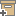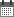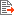ניהול
קהילה:
אסיף מאגר המחקר החקלאי
The dual probe heat pulse method: interaction between probes of finite radius and finite heat capacityBack to searchPrevious item
Next item
Year:
2016
Source of publication :
Journal of Engineering Mathematics
Authors :
קמאי, תמיר
;
.
Volume :
99
Co-Authors:
We analyze the dual-probe heat-pulse (DPHP) method for measuring the thermal properties of soil or other media. The method involves measuring the temperature rise of a receiver probe that is parallel to, and a known distance from, an emitter probe from which a pulse of heat is released. Under the assumption that the probes are perfect conductors, we derive a semi-analytical solution for this method that accounts for the finite radius and finite conductivity of the probes and contact resistance between probe and soil. The solution in the Laplace domain is obtained by writing solutions of the Helmholtz equation around each probe as infinite series of terms involving Bessel and trigonometric functions. Addition theorems are then used to write the solutions centred at each probe in terms of solutions centred at the other probe. Truncating the series and solving a system of linear equations gives numerical values for the series coefficients, which in turn gives values of the Laplace transforms for numerical inversion. We use the solution to investigate the validity of a simpler approximate solution that is being used in conjunction with the DPHP method for thermal property determination. For what we define as typical implementations of the method, our results show that error resulting from use of the approximate solution is sufficiently small that its effect on estimated thermal properties will be negligible. The same general approach can be used to investigate a growing number of DPHP applications for which the approximate solution may be of questionable accuracy. © 2015, Springer Science+Business Media Dordrecht.
Facilitators :
From page:
79
To page:
102
(
Total pages:
24
)
Abstract:
Bessel function addition theorems; Dual-probe heat-pulse method; Numerical inverse Laplace transform; Soil thermal properties
Note:
Related Files :
Numerical inverse Laplace transform
Semi-analytical solution
Soils
Soil thermal properties
System of linear equations
עוד תגיות
תוכן קשור
More details
DOI :
Article number:
Affiliations:
Database:
סקופוס
Publication Type:
מאמר
;
.
Language:
אנגלית
Editors' remarks:
ID:
20794
Last updated date:
02/03/2022 17:27
Creation date:
16/04/2018 23:39Scientific Publication
The dual probe heat pulse method: interaction between probes of finite radius and finite heat capacity
99
We analyze the dual-probe heat-pulse (DPHP) method for measuring the thermal properties of soil or other media. The method involves measuring the temperature rise of a receiver probe that is parallel to, and a known distance from, an emitter probe from which a pulse of heat is released. Under the assumption that the probes are perfect conductors, we derive a semi-analytical solution for this method that accounts for the finite radius and finite conductivity of the probes and contact resistance between probe and soil. The solution in the Laplace domain is obtained by writing solutions of the Helmholtz equation around each probe as infinite series of terms involving Bessel and trigonometric functions. Addition theorems are then used to write the solutions centred at each probe in terms of solutions centred at the other probe. Truncating the series and solving a system of linear equations gives numerical values for the series coefficients, which in turn gives values of the Laplace transforms for numerical inversion. We use the solution to investigate the validity of a simpler approximate solution that is being used in conjunction with the DPHP method for thermal property determination. For what we define as typical implementations of the method, our results show that error resulting from use of the approximate solution is sufficiently small that its effect on estimated thermal properties will be negligible. The same general approach can be used to investigate a growing number of DPHP applications for which the approximate solution may be of questionable accuracy. © 2015, Springer Science+Business Media Dordrecht.
The dual probe heat pulse method: interaction between probes of finite radius and finite heat capacity
Bessel function addition theorems; Dual-probe heat-pulse method; Numerical inverse Laplace transform; Soil thermal properties
Scientific Publication
You may also be interested in